# The machine

The annual depreciation of the machine is 10%. After 8 years, the machine is worth 697 euros. What was the price of this machine at the time of purchase?

a1 =  1619.17 Eur

### Step-by-step explanation: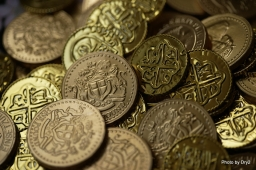Did you find an error or inaccuracy? Feel free to write us. Thank you!Tips to related online calculators
Do you want to convert time units like minutes to seconds?

## Related math problems and questions:

• Machine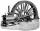Price of the new machine is € 62000. Every year is depreciated 15% of residual value. What will be the value of the machine after 3 years?
• Railways 3Railway Corporation wants to purchase a new machine for $360,000. Management predicts that the machine can produce sales of$220,000 each year for the next 5 years. Expenses are expected to include direct materials, direct labor, and factory overhead (exc
• DepositIf you deposit 719 euros at the beginning of each year, how much money we have at 1.3% (compound) interest after 9 years?
• Annual interest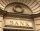A loan of 10 000 euro is to be repaid in annual payments over 10 years. Assuming a fixed 10% annual interest rate compounded annually, calculate: (a) the amount of each annual repayment (b) the total interest paid.
• Washing machineThe washing machine cost € 325. In sale-off, they became cheaper by 10%, later by another 10%. Find the last price of the washing machine.A radioactive material loses 10% of its mass each year. What proportion will be left there after n=6 years?
• Volume of woodEvery year, at the same time, an increase in the volume of wood in the forest is measured. The increase is regularly p% compared to the previous year. If in 10 years the volume of wood has increased by 10%, what is the number p?
• Investment1000\$ is invested at 10% compound interest. What factor is the capital multiplied by each year? How much will be there after n=12 years?
• SaleIf the product twice price cut by 25%, what percentage was price cut in total?
• SavingsThe depositor regularly wants to invest the same amount of money in the financial institution at the beginning of the year and wants to save 10,000 euros at the end of the tenth year. What amount should he deposit if the annual interest rate for the annua
• The city 2Today lives 298000 citizens in the city. How many citizens can we expect in 8 years if their annual increase is 2.4%?
• Ice skates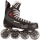Ice skates were raised twice, the first time by 25%, the second time by 10%. After the second price, their cost was 82.5 euros. What was the original price of skates?
• The town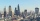The town population is 56000. It is decreasing by 2% every year. What will be the population of the town after 13 years?
• Rising priceThe book first become more expensive by 5 euros. New price was later increased by 8%. After the second price increase book cost 46 euros. What was the original price of the book?
• PopulationWhat is the population of the city with 3% annual growth, if in 10 years the city will have 60,000 residents?
• Geography testsOn three 150-point geography tests, you earned grades of 88%, 94%, and 90%. The final test is worth 250 points. What percent do you need on the final to earn 93% of the total points on all tests?
• InflationOnce upon a time, tsar owned a money printer and printed and printed. The result of printing money prices went up,in the first year 3.9 %, in the second 6%, in the third 4.7% and in the fourth 5.5%. Then tsar was failed in election. Calculate the average